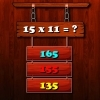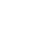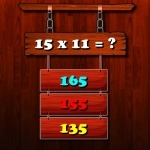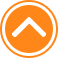×

PlayMultiplication Math Challenge80%Device: 💻 Pc   📱 Mobile
Rating: 80%
🎲Multiplication Math Challenge

Multiplication Math Challenge is an online game that you can play for free. Multiplication Math Challenge is a game of multiplication. You have 10 seconds for each questions. Your brain needs to think quickly to find the right answer and get points. Have a good time. Here you can play Multiplication Math Challenge game and watch its walkthrough for free in friv2020.com. Multiplication Math Challenge is an amazing game from our list of Friv 2020 games.

🎮 Instructions

Use Left Mouse button to Play

📂 Categories

thinking, arcade, puzzle, math, mathka, numbers, school, skill, skills, mathematical, mathematics, multiplication, mathematic, brain, ..

👌 Recommended
📌 Tags